# Estimating sigma

Sigma (σ) is the standard deviation of the process. If you enter an historical value for σ, then Minitab uses the historical value. Otherwise, Minitab uses one of the following methods to estimate σ from the data.

## Average moving range method

The average moving range,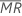, of length w is given by the following formula:

where MRi is the moving range for observation i, calculated as follows:

Minitab usesto calculate Smr, which is an unbiased estimate of σ:

### Notation

TermDescription
nnumber of observations
w length of the moving range. The default is 2.
d2() value of unbiasing constant d2 that corresponds to the value specified in parentheses.

## Median moving range method

The median moving range,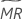, of length w is given by the following formula:

where MRi is the moving range for observation i, calculated as follows:

Minitab usesto calculate Smr, which is an unbiased estimate of σ:

### Notation

TermDescription
nnumber of observations
w length of the moving range. The default is 2.
d4() value of unbiasing constant d4 that corresponds to the value specified in parentheses.

## Square root of MSSD method

MSSD stands for the mean of squared successive differences. The square root of the MSSD (SRMSSD) is calculated as follows:

### Notation

TermDescription
didifference between the value of observation i and the value of observation i – 1
Nnumber of observations
c4'(N)unbiasing constant from a table

## Rbar method

Minitab uses the range of each subgroup,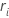, to calculate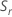, which is an unbiased estimator of σ:

where

When the subgroup size is constant, the formula simplifies to the following:

where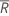(Rbar) is the mean of the subgroup ranges, calculated as follows:

### Notation

TermDescription
rirange for subgroup i
m number of subgroups
d2(·)value of unbiasing constant d2 that corresponds to the value specified in parentheses.
ninumber of observations in subgroup i
d3(·)value of unbiasing constant d3 that corresponds to the value specified in parentheses.

## Sbar method

### Without unbiasing constant

If you do not use an unbiasing constant, then the Sbar is the mean of the subgroup standard deviations:

### With unbiasing constant

If you use the unbiasing constant, c4(ni), then Sbar is calculated as follows:

When the subgroup size is constant, Sbar is:

### Notation

TermDescription
c4 (ni)value of the unbiasing constant c4 that corresponds to the value that is specified in parentheses.
Sistandard deviation of subgroup i
mnumber of subgroups

## Pooled standard deviation method

The pooled standard deviation (Sp) is given by the following formula:

When the subgroup size is constant, Sp can also be calculated as follows:

### With unbiasing constant

By default, Minitab applies the unbiasing constant, c4(), when you use the pooled standard deviation to estimate σ:

When the subgroup size is constant, the unbiased Sp can also be calculated as follows:

### Notation

TermDescription
xijjth observation in the ith subgroup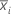mean of subgroup i
ninumber of observations in subgroup i
μvmean of the subgroup variances
c4(·)value of the unbiasing constant c4 that corresponds to the value that is specified in parentheses.
ddegrees of freedom for Sp, given by the following formula: